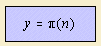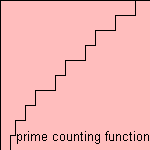# prime counting function

## discrete curveThe prime counting function π(n) gives the number of primes equal or less than n.

According the Prime Number Theorem 1), for large n the function is approximated by the logarithmic prime counting approximation.

Better approximations of the function have been found as:

notes

1) Ash 1971 p. 196.